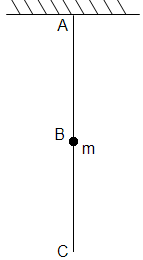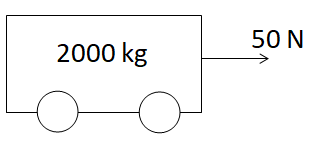In the  Question, if the string C is stretched slowly, then(1) The portion AB of the string will break

(2) The portion BC of the string will break

(3) None of the strings will break

(4) None of the above

Concept Questions :-

Newton laws
High Yielding Test Series + Question Bank - NEET 2020

Difficulty Level:

Newton's second law gives the measure of

(1) Acceleration

(2) Force

(3) Momentum

(4) Angular momentum

Concept Questions :-

Newton laws
High Yielding Test Series + Question Bank - NEET 2020

Difficulty Level:

A coin is dropped in a lift. It takes time t1 to reach the floor when lift is stationary. It takes time t2 when lift is moving up with constant acceleration. Then

(1) t1 > t2

(2) t2 > t1

(3) t1 = t2

(4) t1 >> t2

Concept Questions :-

Application of laws
High Yielding Test Series + Question Bank - NEET 2020

Difficulty Level:

If the tension in the cable of 1000 kg elevator is 1000 kg weight, the elevator

(1) Is accelerating upwards

(2) Is accelerating downwards

(3) May be at rest or accelerating

(4) May be at rest or in uniform motion

Concept Questions :-

Newton laws
High Yielding Test Series + Question Bank - NEET 2020

Difficulty Level:

An object with a mass 10 kg moves at a constant velocity of 10 m/sec. A constant force then acts for 4 second on the object and gives it a speed of 2 m/sec in opposite direction. The acceleration produced in it, is

(1) 3 m/sec2

(2) –3 m/sec2

(3) 0.3 m/sec2

(4) –0.3 m/sec2

Concept Questions :-

Newton laws
High Yielding Test Series + Question Bank - NEET 2020

Difficulty Level:

The force acting on the object of mass 1 kg moving with acceleration $3m/{s}^{2}$ is -

(1) 30 N

(2) – 30 N

(3) 3 N

(4) – 3 N

Concept Questions :-

Newton laws
High Yielding Test Series + Question Bank - NEET 2020

Difficulty Level:

A machine gun is mounted on a 2000 kg car on a horizontal frictionless surface. At some instant the gun fires bullets of mass 10 gm with a velocity of 500 m/sec with respect to the car. The number of bullets fired per second is ten. The average thrust on the system is

(1) 550 N

(2) 50 N

(3) 250 N

(4) 250 dyne

Concept Questions :-

Newton laws
High Yielding Test Series + Question Bank - NEET 2020

Difficulty Level:In the above diagram, the acceleration of the car will be

(1) 0.25 m/sec2

(2) 2.5 m/sec2

(3) 5.0 m/sec2

(4) 0.025 m/sec2

Concept Questions :-

Application of laws
High Yielding Test Series + Question Bank - NEET 2020

Difficulty Level:

A particle of mass 0.3 kg is subjected to a force F = –kx with k = 15 N/m. What will be its initial acceleration if it is released from a point 20 cm away from the origin

(1) 5 m/s2

(2) 10 m/s2

(3) 3 m/s2

(4) 15 m/s2

Concept Questions :-

Spring force
High Yielding Test Series + Question Bank - NEET 2020

Difficulty Level:

An elevator weighing 6000 kg is pulled upward by a cable with an acceleration of 5 ms–2. Taking g to be 10 ms–2, then the tension in the cable is

(1) 6000 N

(2) 9000 N

(3) 60000 N

(4) 90000 N

Concept Questions :-

Application of laws